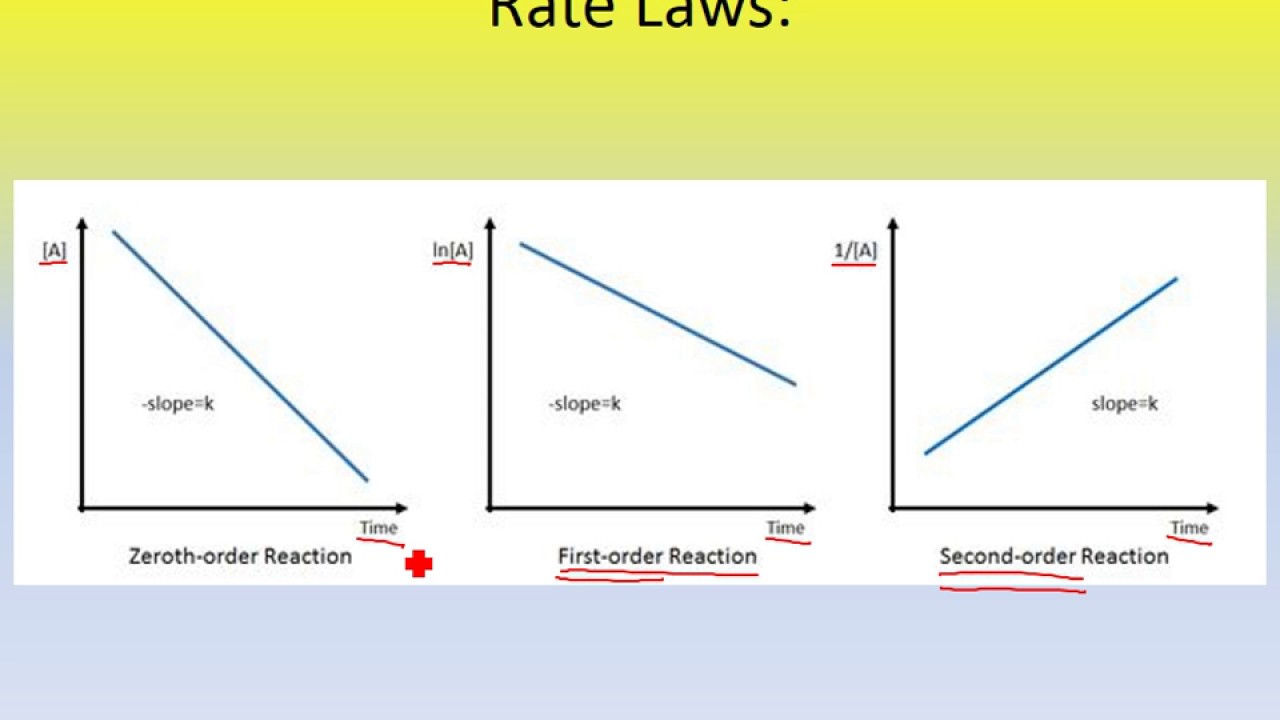## Order

Rhea Shah 2F
Posts: 97
Joined: Thu Jul 25, 2019 12:17 am

### Order

How do you know which order rate law to use for a reaction?

Juliana Chopelas 1A
Posts: 50
Joined: Sat Aug 24, 2019 12:16 am

### Re: Order

You can look at the reactions graph. Which ever graph produces a straight line will give you the reaction order. For zero order the graph would be (A) v time and would give a straight line with a -slope. First order reaction would be ln(A) v time and would give a straight line with a -slope. Second order would be 1/(A) v time and would give a straight line with a +slope.

205154661_Dis2J
Posts: 109
Joined: Wed Sep 18, 2019 12:21 am

### Re: Order

You can look at the graphs provided or you can look at the units used in a specific problem if they do not specifically tell you which order it is. That is why it is important to know and memorize the units for the orders!

Eva Zhao 4I
Posts: 101
Joined: Sun Sep 29, 2019 12:16 am

### Re: Order

Here is a diagram of the graphs mentioned above, in case you might find it helpful:805097738
Posts: 180
Joined: Wed Sep 18, 2019 12:20 am

### Re: Order

Juliana Chopelas 1A wrote:You can look at the reactions graph. Which ever graph produces a straight line will give you the reaction order. For zero order the graph would be (A) v time and would give a straight line with a -slope. First order reaction would be ln(A) v time and would give a straight line with a -slope. Second order would be 1/(A) v time and would give a straight line with a +slope.

are these graphs always labeled to clearly see that? because the line for zero and first both have -slope

Jack Riley 4f
Posts: 100
Joined: Sat Aug 24, 2019 12:17 am

### Re: Order

you look at the graph and compare it with the 3 possible orders to determine which one best fits the graph shown